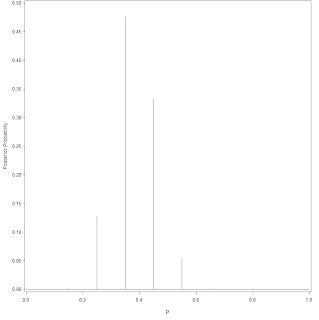by sxlion

SAS绘图即学即用系列连载4.1 –曲线图： 以下代码 可以拷贝到SAS编辑器中，直接使用；稍作更改便可得到自己满意的图形。以下来自一本关于SAS绘图的书稿（未出版草稿），均为本人原创。 完整代码详见SAS资源资讯列表  www.saslist.net

4.1  线图

4.11 曲线图123456789 FILENAME file "d:SAS_chartssample411.png"; goptions reset=all device=png gsfname=file/*设置图片格式和存放点*/ hsize=8cm vsize=6cm ; /* 设置绘图区域大小 */ symbol value=none interpol=j CI=orange width=3; /* 设置线点属性 */ proc gplot data=sashelp.stocks; plot high*date; where stock="IBM" and ('01feb90'd <= date <= '01feb91'd); run; quit;

4.12 分组曲线图1234567891011 FILENAME file "d:SAS_chartssample413.png"; goptions reset=all device=png gsfname=file/*设置图片格式和存放点*/ hsize=8cm vsize=6cm ; /* 设置绘图区域大小 */ symbol1 value=none interpol=join CI=red width=2; symbol2 value=none interpol=join CI=blue width=2; symbol3 value=none interpol=join CI=orange width=2; proc gplot data=sashelp.stocks; plot high*date=stock; where ('01feb90'd < date < '01feb91'd); run; quit;

4.13 双/多曲线图1234567891011 FILENAME file "d:SAS_chartssample414.png"; goptions reset=all device=png gsfname=file/*设置图片格式和存放点*/ hsize=10cm vsize=8cm ; /* 设置绘图区域大小 */ symbol1 value=none interpol=join CI=red L=2 width=1; /*L:设置线型 */ symbol2 value=none interpol=join CI=orange L=1 width=2; symbol3 value=none interpol=join CI=green L=3 width=1; proc gplot data=sashelp.stocks; plot high*date close *date low*date /overlay ; where stock="IBM" and ('01feb90'd < date < '01feb91'd); run; quit;

4.14 双坐标曲线图1234567891011 FILENAME file "d:SAS_chartssample415.png"; goptions reset=all device=png gsfname=file/*设置图片格式和存放点*/ hsize=10cm vsize=8cm ; /* 设置绘图区域大小 */ symbol value=none interpol=j CI=red width=1; /* 设置线属性 */ symbol2 value=none interpol=j CI=black width=1; /* 设置点属性 */ proc gplot data=sashelp.stocks; plot high*date/vzero; plot2 volume*date; where stock="IBM" and ('01feb90'd <= date <= '01feb91'd); run; quit;

4.15  其他修饰：1234567891011121314151617181920 FILENAME file "d:SAS_chartssample416.png"; goptions reset=all device=png gsfname=file/*设置图片格式和存放点*/ hsize=12cm vsize=8cm ; /* 设置绘图区域大小 */ symbol1 value=none interpol=join CI=red width=2;/* 设置点属性 */ symbol2 value=none interpol=join CI=orange width=2; symbol3 value=none interpol=join CI=green width=2; axis1 order= ('01jan86'd to '01jan06'd by 1461) LABEL=( "Period") minor=none offset=(0.2 cm); /*调整坐标轴的显示、改变轴标签内容*/ axis2 order= (0 to 250 by 50) LABEL=(angle=90 "") major=none minor=none; /*调整坐标轴的显示、改变轴标签内容和位置*/ legend1 label=none position=(top right inside) mode=share; Title H=0.4cm "Three company's stock from '01JAN86' to '01JAN06' "; Footnote justify=left "Data resource: sashelp.stocks" ; proc gplot data=sashelp.stocks; plot close*date=stock / haxis=axis1 vaxis=axis2 vref=50 to 200 by 50 lvref=1 CVREF=black WVREF=1 legend=legend1 ; run; quit;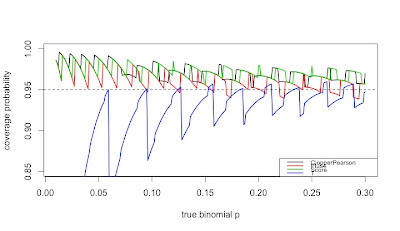While traditional statistics courses teach students to calculate intervals and test for binomial proportions using a normal or t approximation, this method does not always work well. Agresti and Coull ("Approximate is better than "exact' for interval estimation of binomial proportions". The American Statistician, 1998; 52:119-126) demonstrated this and reintroduced an improved (Plus4) estimator originally due to Wilson (1927).

In this entry, we demonstrate how the coverage varies as a function of the underlying probability and compare four intervals: (1) t interval, (2) Clopper-Pearson, (3) Plus4 (Wilson/Agresti/Coull) and (4) Score, using code contributed by Albyn Jones from Reed College. Here, coverage probability is defined as the expected value that a CI based on an observed value will cover the true binomial parameter that generated that value. The code calculates the coverage probability as a function of a given binomial probability p and a sample size n. Intervals are created for each of the possible outcomes from 0, ..., n, then checked to see if the intervals include the true value. Finally, the sum of the probabilities of observing outcomes in which the binomial parameter is included in the interval determines the exact coverage. Note several distinct probabilities: (1) binomial parameter "p", the probability of success on a trial; (2) probability of observing x successes in N trials, P(X=x); (3) coverage probability as defined above. For distribution quantiles and probabilities, see section 1.10 and table 1.1.

R

We begin by defining the support functions which will be used to calculate the coverage probabilities for a specific probability and sample size.
`CICoverage = function(n, p, alpha=.05) {  # set up a table to hold the results  Cover = matrix(0, nrow=n+1, ncol=5)  colnames(Cover)=c("X","ClopperPearson","Plus4","Score","T")  Cover[,1]=0:n  zq = qnorm(1-alpha/2)  tq = qt(1-alpha/2,n-1)  for (i in 0:n) {    Phat = i/n    P4 = (i+2)/(n+4)    # Calculate T and plus4 intervals manually,     # use canned functions for the other     TInt = Phat + c(-1,1)*tq*sqrt(Phat*(1-Phat)/n)    P4Int = P4 + c(-1,1)*zq*sqrt(P4*(1-P4)/(n+4))    CPInt= binom.test(i,n)\$conf.int    SInt = prop.test(i,n)\$conf.int    # check to see if the binomial p is in each CI     Cover[i+1,2] = InInt(p, CPInt)    Cover[i+1,3] = InInt(p, P4Int)    Cover[i+1,4] = InInt(p, SInt)    Cover[i+1,5] = InInt(p, TInt)  }  # probability that X=x   p = dbinom(0:n, n, p)  ProbCover=rep(0, 4)  names(ProbCover) = c("ClopperPearson", "Plus4", "Score", "T")  # sum P(X=x) * I(p in CI from x)  for (i in 1:4){    ProbCover[i] = sum(p*Cover[,i+1])  }  list(n=n, p=p, Cover=Cover, PC=ProbCover)}`

In addition, we define a function to determine whether something is in the interval.
`InInt = function(p,interval){  interval <= p && interval >= p}`

Finally, there's a function which summarizes the results.
`CISummary = function(n, p) {  M = matrix(0,nrow=length(n)*length(p),ncol=6)  colnames(M) = c("n","p","ClopperPearson","Plus4","Score","T")  k=0  for (N in n) {    for (P in p) {      k=k+1      M[k,]=c(N, P, CICoverage(N, P)\$PC)    }  }  data.frame(M)}`

We then generate the CI coverage plot provided at the start of the entry, which uses sample size n=50 across a variety of probabilities.
`lwdval = 2nvals = 50probvals = seq(.01, .30, by=.001)results = CISummary(nvals, probvals)plot(range(probvals), c(0.85, 1), type="n", xlab="true binomial p",     ylab="coverage probability")abline(h=0.95, lty=2)lines(results\$p, results\$ClopperPearson, col=1, lwd=lwdval)lines(results\$p, results\$Plus4, col=2, lwd=lwdval)lines(results\$p, results\$Score, col=3, lwd=lwdval)lines(results\$p, results\$T, col=4, lwd=lwdval)tests = c("ClopperPearson", "Plus4", "Score", "T")legend("bottomright", legend=tests,       col=1:4, lwd=lwdval, cex=0.70)`

The resulting plot is quite interesting, and demonstrates how non-linear the coverage is for these methods, and how the t (almost equivalent to the normal, in this case) is anti-conservative in many cases. It also confirms the results of Agresti and Coull, who concluded that for interval estimation of a proportion, coverage probabilities from inverting the standard binomial and too small when inverting the Wald large-sample normal test, with the Plus 4 yielding coverage probabilities close to the desired, even for very small sample sizes.

SAS
Calculating the coverage probability for a given N and binomial p can be done in a single data step, summing the probability-weighted coverage indicators over the realized values of the random variate. Once this machinery is developed, we can call it repeatedly, using a macro, to find the results for different binomial p. We comment on the code internally.
`%macro onep(n=,p=,alpha=.05);data onep;n = &n;p = &p;alpha = α/* set up collectors of the weighted coverage indicators */expcontrib_t = 0;expcontrib_p4 = 0;expcontrib_s = 0;expcontrib_cp = 0;/* loop through the possible observed successes x*/do x = 0 to n;  probobs = pdf('BINOM',x,p,n);  /* probability X=x */  phat = x/n;  zquant = quantile('NORMAl', 1 - alpha/2, 0, 1);  p4 = (x+2)/(n + 4);  /* calculate the half-width of the t and plus4 intervals */  thalf = quantile('T', 1 - alpha/2,(n-1)) * sqrt(phat*(1-phat)/n);  p4half = zquant * sqrt(p4*(1-p4)/(n+4));  /* the score CI in R uses a Yates correction by default, and is      reproduced here */  yates = min(0.5, abs(x - (n*p)));  z22n = (zquant**2)/(2*n);  yl = phat-yates/n;  yu = phat+yates/n;  slower = (yl + z22n - zquant * sqrt( (yl*(1-yl)/n) + z22n / (2*n) )) /    (1 + 2 * z22n);   supper = (yu + z22n + zquant * sqrt( (yu*(1-yu)/n) + z22n / (2*n) )) /    (1 + 2 * z22n);   /* cover = 1 if in the CI, 0 else */  cover_t = ((phat - thalf) < p) and ((phat + thalf) > p);  cover_p4 = ((p4 - p4half) < p) and ((p4 + p4half) > p);  cover_s = (slower < p) and (supper > p);  /* the Clopper-Pearson interval can be easily calculated on the fly */   cover_cp = (quantile('BETA', alpha/2 ,x,n-x+1) < p) and     (quantile('BETA', 1 - alpha/2 ,x+1,n-x) > p);   /* cumulate the weighted probabilities */  expcontrib_t = expcontrib_t + probobs * cover_t;  expcontrib_p4 = expcontrib_p4 + probobs * cover_p4;  expcontrib_s = expcontrib_s + probobs * cover_s;  expcontrib_cp = expcontrib_cp + probobs * cover_cp;  /* only save the last interation */  if x = N then output;  end;run;%mend onep;`

The following macro calls the first one for a series of binomial p for a fixed N. Since the macro %do loop can only iterate through integers, we have to do a little division; the %sysevelf function will do this within the macro.
`%macro repcicov(n=, lop=, hip=, byp=, alpha= .05);/* need an empty data set to store the results */data summ; set _null_; run;%do stepp = %sysevalf(&lop / &byp, floor) %to %sysevalf(&hip / &byp,floor);  /* note that the p sent to the %onep macro is a                            text string like "49 * .001" */  %onep(n = &n, p = &stepp * &byp, alpha = &alpha);  /* tack on the current results to the ones finished so far */  /* this is a simple but inefficient way to add each binomial p into      the output data set */  data summ; set summ onep; run;%end;%mend repcicov;/* same parameters as in R */%repcicov(n=50, lop = .01, hip = .3, byp = .001);`

Finally, we can plot the results. One option shown here and not mentioned in the book are the mode=include option to the symbol statement, which allows the two distinct pieces of the T coverage to display correctly.
`goptions reset=all;legend1 label=none position=(bottom right inside)        mode=share across=1 frame value = (h=2);axis1 order = (0.85 to 1 by 0.05) minor=none    label = (a=90 h=2 "Coverage probability") value=(h=2);axis2 order = (0 to 0.3 by 0.05) minor=none    label = (h=2 "True binomial p") value=(h=2);symbol1 i = j v = none l =1 w=3 c=blue mode=include;symbol2 i = j v = none l =1 w=3 c=red;symbol3 i = j v = none l =1 w=3 c=lightgreen;symbol4 i = j v = none l =1 w=3 c=black;proc gplot data = summ;plot (expcontrib_t expcontrib_p4  expcontrib_s  expcontrib_cp) * p   / overlay legend vaxis = axis1 haxis = axis2 vref = 0.95 legend = legend1;label expcontrib_t = "T approximation" expcontrib_p4 = "P4 method"      expcontrib_s = "Score method" expcontrib_cp = "Exact (CP)";run; quit;`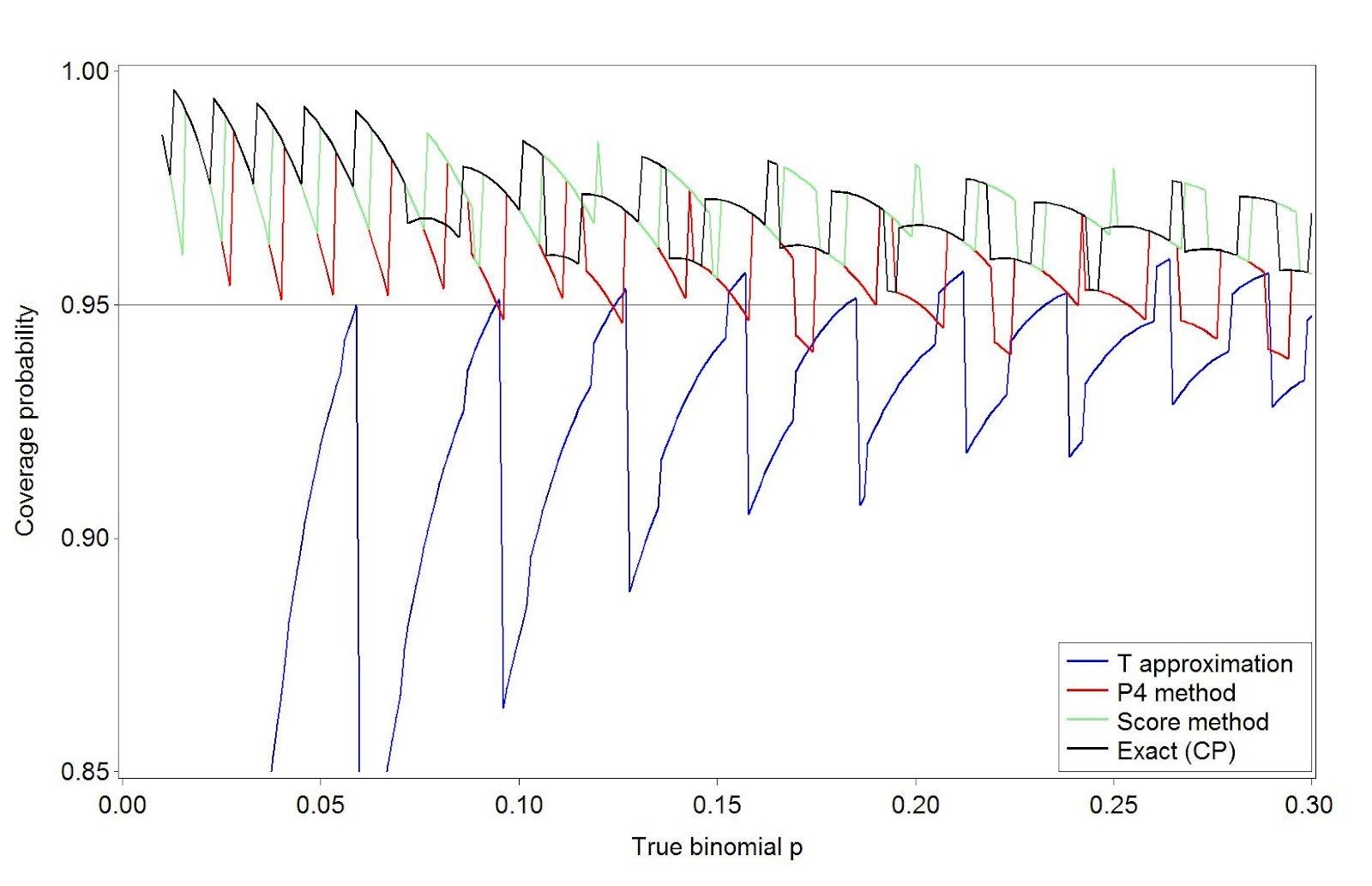An unrelated note about aggregators:We love aggregators! Aggregators collect blogs that have similar coverage for the convenience of readers, and for blog authors they offer a way to reach new audiences. SAS and R is aggregated by R-bloggers, PROC-X, and statsblogs with our permission, and by at least 2 other aggregating services which have never contacted us. If you read this on an aggregator that does not credit the blogs it incorporates, please come visit us at SAS and R. We answer comments there and offer direct subscriptions if you like our content. In addition, no one is allowed to profit by this work under our license; if you see advertisements on this page, the aggregator is violating the terms by which we publish our work.Robert Rutledge's book Just Enough SAS is the source of this week's SAS tip. It would be easy to turn almost any page of Robert's book into a stand-alone tip. However, today my attention was drawn to two side-by-side pages. One features a PROC GCHART pie chart (and includes a DONUT statement) and the other [...]A colleague recently asked "why should the average get closer to the mean when we increase the sample size?" We should interpret this question as asking why the standard error of the mean gets smaller as n increases. The central limit theorem shows that (under certain conditions, of course) the standard error must do this, and that the mean approaches a normal distribution. But the question was why does it? This seems so natural that it may have gone unquestioned in the past.

The best simple rationale may be that there are more ways to get middle values than extreme values--for example, the mean of a die roll (uniform discrete distribution on 1, 2, ..., 6) is 3.5. With one die, you're equally likely to get an "average" of 3 or of 1. But with two dice there are five ways to get an average of 3, and only one way to get an average of 1. You're 5 times more likely to get the value that's closer to the mean than the one that's further away.

Here's a simple graphic to show that the standard error decreases with increasing n.

SAS
We begin by simulating some data-- normal, here, but of course that doesn't matter (assuming that the standard deviation exists for whatever distribution we pick and the sample size is appropriately large). Rather than simulate separate samples with n = 1 ... k, it's easier to add a random variate to a series and keep a running tally of the mean, which is easy with a little algebra. This approach also allows tracking the progress of the mean of each series, which could also be useful.

`%let nsamp = 100;data normal;do sample = 1 to &nsamp;  meanx = 0;  do obs = 1 to &nsamp;    x = normal(0); meanx = ((meanx * (obs -1)) + x)/obs; output;  end;end;run;`

We can now plot the means vs. the number of observations.
`symbol1 i = none v = dot h = .2;proc gplot data = normal;plot meanx * obs;run;quit;symbol1 i=join v=none r=&nsamp;proc gplot data=normal;  plot meanx * obs = sample / nolegend;run; quit;`

The graphic resulting from the first proc gplot is shown above, and demonstrates both how quickly the variability of the estimate of the mean decreases when n is small, and how little it changes when n is larger. A plot showing the means for each sequence converging can be generated with the second block of code. Note the use of the global macro variable nsamp assigned using the %let statement (section A.8.2).

R
We'll also generate sequences of variates in R. We'll do this by putting the random variates in a matrix, and treating each row as a sequence. We'll use the apply() function (sections 1.10.6 and B.5.3) to treat each row of the matrix separately.
`numsim = 100matx = matrix(rnorm(numsim^2), nrow=numsim)runavg = function(x) { cumsum(x)/(1:length(x)) }ramatx = t(apply(matx, 1, runavg))`

The simple function runavg() calculates the running average of a vector and returns the a vector of equal length. By using it as the function in apply() we can get the running average of each row. The result must be transposed (with the t() function, section 1.9.2) to keep the sequences in rows. To plot the values, we'll use the type="n" option to plot(), specifying the first column of the running total as the y variable. While it's possible that the running average will surpass the average when n=1, we ignore that case in this simple demonstration.
`plot(x=1:numsim, y = ramatx[,1], type="n",  xlab="number of observations", ylab="running mean")rapoints = function(x) points(x~seq(1:length(x)), pch=20, cex=0.2)apply(ramatx,1,rapoints)plot(x=1:numsim, y = ramatx[,1], type="n",  xlab="number of observations", ylab="running mean")ralines = function(x) lines(x~seq(1:length(x)))apply(ramatx, 1, ralines)`

Here we define another simple function to plot the values in a vector against the place number, then again use the apply() function to plot each row as a vector. The first set of code generates a plot resembling the SAS graphic presented above. The second set of code will connect the values in each sequence, with results shown below.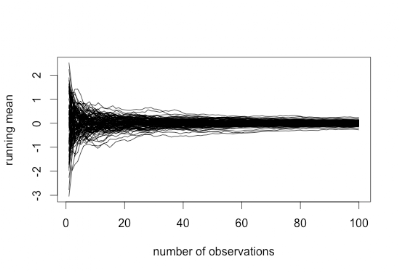A facebook friend posted the picture reproduced above-- it makes the case that President Obama has been a successful creator of jobs, and also paints GW Bush as a president who lost jobs. Another friend pointed out that to be fair, all of Bush's presidency ought to be included. Let's make a fair plot of job growth and loss. Data can be retrieved from the Bureau of Labor Statistics, where Nick will be spending his next sabbatical. The extract we use below is also available from the book website. This particular table reports the cumulative change over the past three months, adjusting for seasonal trends. This tends to smooth out the line.

SAS

The first job is to get the data into SAS. Here we demonstrate reading it directly from a URL, as outlined in section 1.1.6.
`filename myurl   url "http://www.math.smith.edu/sasr/datasets/bls.csv";       data q_change;   infile myurl delimiter=',';input  Year Jan Feb Mar Apr May Jun Jul Aug Sep Oct Nov Dec Annual;run;`

The raw data are in a pretty inconvenient format for plotting. To make a long, narrow data set with a row for each month, we'll use proc transpose (section 1.5.3) to flip each year on its side. Then, to attach a date to each measure, we'll use the compress function. First we add "01" (the first of the month) to the month name, which is in a variable created by proc transpose with the default name "_name_". Then we tack on the year variable, and input the string in the date format. The resulting variable is a SAS date (number of days since 12/31/1959, see section 1.6.1).
`proc transpose data=q_change out=q2;  by year;run;data q3;set q2;  date1 = input(compress("01"||_name_||year),date11.);run;`

Now the data are ready to plot. It would probably be possible to use proc sgplot but proc gplot is more flexible and allows better control for presentation graphics.
`title "3-month change in private-sector jobs, seasonally adjusted";axis1 minor = none label = (h=2 angle = 90 "Thousands of jobs")  value = (h = 2);axis2 minor = none value = (h=2)label = none   offset = (1cm, -5cm)  reflabel = (h=1.5 "Truman" "Eisenhower" "Kennedy/Johnson"     "Nixon/Ford" "Carter" "Reagan" "GHW Bush" "Clinton" "GW Bush" "Obama" );symbol1 i=j v=none w=3;proc gplot data=q3;plot col1 * date1 / vaxis=axis1 haxis=axis2 vref=0   href = '12apr1945'd '21jan1953'd '20jan1961'd '20jan1969'd     '20jan1977'd '21jan1981'd '20jan1989'd '20jan1993'd     '20jan2001'd '20jan2009'd;format date1 monyy6.;run;quit;`

Much of the syntax above has been demonstrated in our book examples and blog entries. What may be unfamiliar is the use of the href option in the plot statement and the reflabel option in the axis statement. The former draws reference lines at the listed values in the plot, while the latter adds titles to these lines. The resulting plot is shown here.Looking fairly across postwar presidencies, only the Kennedy/Johnson and Clinton years were mostly unmarred by periods with large losses in jobs. The Carter years were also times jobs were consistently added. While the graphic shared on facebook overstates the case against GW Bush, it fairly shows Obama as a job creator thus far, to the extent a president can be credited with jobs created on his watch.

R

The main trick in R is loading the data and getting it into the correct format.
here we use cbind() to grab the appropriate columns, then transpose that matrix and turn it into a vector which serves as input for making a time series object with the ts() command (as in section 4.2.8). Once this is created, the default plot for a time series object is close to what we have in mind.
`ds = read.csv("http://www.math.smith.edu/sasr/datasets/bls.csv",               header=FALSE)jobs = with(ds, cbind(V2, V3, V4, V5, V6, V7, V8, V9, V10,                      V11, V12, V13))jobsts = ts(as.vector(t(jobs)), start=c(1945, 1),             frequency=12)plot(jobsts, plot.type="single", col=4,     ylab="number of jobs (in thousands)")`

All that remains is to add the reference lines for 0 jobs and the presidencies. The lines are most easily added with the abline() function (section 5.2.1). Easier than adding labels for the lines within the plot function will be to use the mtext() function to place the labels in the margins. We'll write a little function to save a few keystrokes by plotting the line and adding the label together.
`abline(h=0)presline = function(date,line,name){  mtext(at=date,text= name, line=line)  abline(v = date)}presline(1946,1,"Truman")presline(1953,2,"Eisenhower")presline(1961,1,"Kennedy/Johnson")presline(1969,2,"Nixon/Ford")presline(1977,1,"Carter")presline(1981,2,"Reagan")presline(1989,1,"GHW Bush")presline(1993,2,"Clinton")presline(2001,1,"GW Bush")presline(2009,2,"Obama")`

It might be worthwhile to standardize the number of jobs to the population size, since the dramatic loss of jobs due to demobilization after the Second World War during a single month in 1945 (2.4 million) represented 1.7% of the population, while the recent loss of 2.3 million jobs in 2009 represented only 0.8% of the population.this pdf file list different colors used in SAS.

/* Set the graphics environment */
goptions reset=all cback=white border htitle=12pt;

/* Create input data set, PRODCOST */
data prodcost (drop=tcprev tfc tvc tc);
retain tcprev 0;
input quan tfc tvc;
tc=tfc+tvc;
afc=tfc/quan;       /* average fixed cost    */
avc=tvc/quan;       /* average variable cost */
atc=tc/quan;        /* average total cost    */
mc=tc-tcprev;       /* marginal cost         */
tcprev=tc;
datalines;
1    10   05
2    10   08
3    10   10
4    10   11
5    10   13
6    10   16
7    10   20
8    10   25
9    10   31
10   10   38
11   10   46
;
run;

data anno;
set prodcost end=last;
function='label';
retain xsys ysys '2' hsys '9' position '6';
size=3;
if last then do;
x=quan;
y=afc; color='depk'; text='AFC'; output;
y=avc; color='vibg'; text='AVC'; output;
y=atc; color='mob';  text='ATC'; output;
y=mc;  color='black'; text='MC'; output;
end;
run;

/* Create axis definitions */
axis1 minor=none offset=(1,8)
label=('Thousands of Units');
axis2 order=(0 to 16 by 4) minor=(number=3 color=red height=.3 width=5) offset=(0,0)
label=('Dollar Cost' justify=left '(in hundreds)');

symbol1 interpol=spline color=depk width=1;
symbol2 interpol=spline color=vibg width=1;
symbol3 interpol=spline color=mob  width=1;
symbol4 interpol=spline color=black width=1;
title1 'Projected cost of production';
proc gplot data=prodcost;
plot (afc avc atc mc)*quan / overlay frame vaxis=axis2 haxis=axis1 annotate=anno;
run;
quit;

/* spline用来连接data成为一条曲线 */
/* overlay用来把图片叠加 */
/* minor用来设置小的刻度(major用来设置大的刻度) */
/* offset用来在坐标轴上起始/末尾位置留下空白 */

/* In this expamle shows how to label part of the data */
/* Using annotate in SAS GPLOT */

goptions reset=all cback=white border htitle=12pt htext=10pt;

/* Set up the annotate data sets */
data anno;
length function colot \$8.;
retain xsys ysys '2' ;
if weight gt 160 then do;
function='label';
x=weight;
y=height;
color='black';
position='2';
text=trim(left(put(height,6.1)));
output;
end;
run;

title1 'Annotate specific points on a graph';

/* Set up symbols and axises */
symbol1 interpol=none value=dot color=vibg h=1.2;
axis1 label=("Weight in pounds") offset=(2,2)pct;
axis2 label=(a=90 "Height in inches") order=(55 to 80 by 5) minor=(n=4);

plot height*weight / haxis=axis1 vaxis=axis2 href=160 lhref=20 chref=depk annotate=anno;
/* href draws the verticle line in the plot */
run;
quit;

***********************************************************************************

goptions reset=all cback=white border htitle=12pt htext=10pt;
/* Set up the annotate data sets */
data anno2;
length function color \$8.;
retain xsys ysys '2' ;
function='symbol';
size=2;
x=weight;
y=height;
text='dot';
if weight gt 160 then color='depk';
else color='vibg';
output;
run;

title1 'Annotate specific points on a graph with diff color';

/* Set up symbols and axises */
symbol1 interpol=none  color=white h=1.2;
axis1 label=("Weight in pounds") offset=(2,2)pct;
axis2 label=(a=90 "Height in inches") order=(55 to 80 by 5) minor=(n=4);

plot height*weight / haxis=axis1 vaxis=axis2 href=160 lhref=20 chref=depk annotate=anno2;
/* href draws the verticle line in the plot */
run;
quit;

o
data crime;
infile 'c:\crime.txt';
input state \$ crime pctmetro;
run;

/* First, we will plot crime v.s. pctmetro */
goptions reset=all;
axis1 label=(a=90 "Crime per 1,000,000");
symbol1 value=star color=red;
proc gplot data=crime;
plot crime*pctmetro;
run;

/* In this plot, we don't know which state does the star mean */
/* Try to list the state names in the graph */
/* Need to use 'pointlabel' */

goptions reset=all;
axis1 label=(a=90 "Crime per 1,000,000");
symbol1 pointlabel=('#state' c=red h=1) value=none;
proc gplot data=crime;
plot crime*pctmetro=1 / vaxis=axis1;
run;
quit;

We can do it in R. To label the points in R, we will use the function textxy (package{calibrate}) to do 2D label plot. The code is:

names(mydata)="state"
names(mydata)="crime"
names(mydata)="pctmetro"

attach(mydata)

plot(mydata\$pctmetro, mydata\$crime)
textxy(mydata\$pctmetro, mydata\$crime, labs=mydata\$state, cx=1, dcol=2)

``p = seq(0.05, 0.95, by = 0.1)prior = c(1, 5.2, 8, 7.2, 4.6, 2.1, 0.7, 0.1, 0, 0)prior = prior/sum(prior)data = c(11, 16)post = pdisc(p, prior, data)round(cbind(p, prior, post),2)library(lattice)PRIOR=data.frame("prior",p,prior)POST=data.frame("posterior",p,post)names(PRIOR)=c("Type","P","Probability")names(POST)=c("Type","P","Probability")data=rbind(PRIOR,POST)xyplot(Probability~P|Type,data=data,layout=c(1,2), type="h",lwd=3,col="black")``

``options fullstimer formchar='|----|+|---+=|-/\<>*'  formdlim='-'  error=3;/***************************************************//*模仿oloolo大牛的程序。把 Bayesian compution with R 中的例子用SAS写出来；原文为oloolo所写；用来练习，写不出来的，就看看oloolo怎么写的；哈哈 :)*//*The following is to draw the graph for the discrete prior;There are ten points that p may take, each with its weight respectively;*/data p;    do p = .05 to .95 by .1;        output;    end;run;data prior;input prior @@;cards;1  5.2  8  7.2  4.6  2.1  .7  .1  0  0;run;data p;    retain sum_prior 0;    do until (eof1);        set prior end = eof1;        sum_prior + prior;    end;    do until (eof2);        merge p prior end = eof2;        prior = prior/sum_prior;        output;    end;    drop sum_prior;run;goptions reset = all;symbol interpol=Needle value=none line=1 color=black;axis1 label = (angle = 90 'Prior Probability') order = (0 to 0.3 by .05) minor = none;axis2 label = ('p') order = (0 to 1 by .2) minor = none;proc gplot data = p;    plot prior*p / vaxis=axis1 haxis=axis2;run;quit;``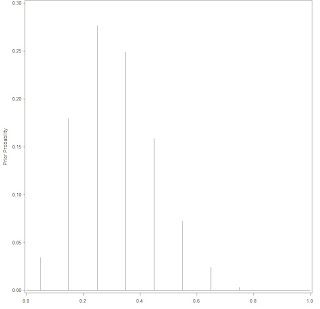``/*Next is to calculate the posterior and then draw it;*/%let n0=16;%let n1=11;/*Calculate the log-likelihood*/data p;   set p; if p > 0 and p < 1 then do;     log_like = log(p)*&n1 + log(1-p)*&n0; end; else     log_like = -999*(p=0)*(&n1 > 0) + (p=1)*(&n0 > 0) ;run;/*Calculate the max(log-likelihood). Since exp(log_likelihood) is very colse to 0, if use it directly, almost allcalculated items is zero. So, use exp(log_likelihood - max), sacle the likelihood. It doesn't change the valueof likelihood since final likelihood = likelihood / sum(likelihood). If both numerator and denominator bothscaled by the same number, it doesn't change the fraction value.*/proc means data = p noprint;    var log_like;    output out = _max  max(log_like)=max;run;data p;    set _max;    sum_like = 0;    do until (eof);        set p end = eof nobs = ntotal;        likelihood = exp(log_like - max);        sum_like + likelihood;    end;    do until (eof1);        set p end = eof1;        likelihood = exp(log_like - max)/sum_like;        posterior = likelihood * prior;        output;    end;run;data p;    sum_post = 0;    do until (eof);        set p end = eof;        sum_post + posterior;    end;    do until (eof2);        set p end =  eof2;        posterior = posterior / sum_post;        output;    end;run;goptions reset = all;symbol interpol=Needle value=none line=1 color=black;axis1 label = (angle = 90 'Posterior Probability') order = (0 to 0.5 by .05) minor = none;axis2 label = ('p') order = (0 to 1 by .2) minor = none;proc gplot data = p;plot posterior*p / vaxis=axis1 haxis=axis2;run;quit;``